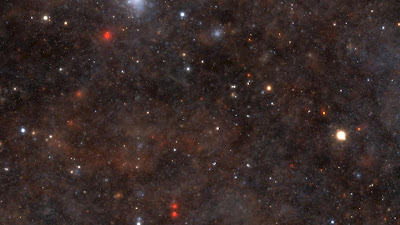I'm preparing assets for an upcoming mini-game jam/hackathon.  My current plan is to make a spaceship video game inspired by Galaxy Trucker, in which you build and then fly a ship.  This post is about the starfield shader that I created by modifying Kali's amazing Star Nest shader on Shadertoy to fit into the game framework.The starfield
The starfield renders in 3.7ms at 1080p on GeForce 650M (the Kepler GPU in a 2012 Macbook Pro) on Windows 7 64-bit, including upsample time.  It is procedural, so it has effectively infinite extent and fine detail down to the pixel level, and has parallax as it scrolls.

I was only willing to spend 25% of the frame time on the background. To keep the performance high, I render downsampled by 2.5x in each direction to an RGB8 texture and then bilinearly interpolate up to full screen resolution.

`// -*- c++ -*-// \file starfield.pix// \author Morgan McGuire//// \cite Based on Star Nest by Kali // https://www.shadertoy.com/view/4dfGDM// That shader and this one are open source under the MIT license//// Assumes an sRGB target (i.e., the output is already encoded to gamma 2.1)#version 120 or 150 compatibility or 420 compatibility#include <compatibility.glsl>// viewport resolution (in pixels)uniform float2    resolution;uniform float2    invResolution;// In the noise-function space. xy corresponds to screen-space XYuniform float3    origin;uniform mat2      rotate;uniform sampler2D oldImage;#define iterations 17#define volsteps 8#define sparsity 0.5  // .4 to .5 (sparse)#define stepsize 0.2#expect zoom #define frequencyVariation   1.3 // 0.5 to 2.0#define brightness 0.0018#define distfading 0.6800void main(void) {        float2 uv = gl_FragCoord.xy * invResolution - 0.5;    uv.y *= resolution.y * invResolution.x;    float3 dir = float3(uv * zoom, 1.0);    dir.xz *= rotate;    float s = 0.1, fade = 0.01;    gl_FragColor.rgb = float3(0.0);        for (int r = 0; r < volsteps; ++r) {        float3 p = origin + dir * (s * 0.5);        p = abs(float3(frequencyVariation) - mod(p, float3(frequencyVariation * 2.0)));        float prevlen = 0.0, a = 0.0;        for (int i = 0; i < iterations; ++i) {            p = abs(p);            p = p * (1.0 / dot(p, p)) + (-sparsity); // the magic formula                        float len = length(p);            a += abs(len - prevlen); // absolute sum of average change            prevlen = len;        }                a *= a * a; // add contrast                // coloring based on distance                gl_FragColor.rgb += (float3(s, s*s, s*s*s) * a * brightness + 1.0) * fade;        fade *= distfading; // distance fading        s += stepsize;    }        gl_FragColor.rgb = min(gl_FragColor.rgb, float3(1.2));    // Detect and suppress flickering single pixels (ignoring the huge gradients that we encounter inside bright areas)    float intensity = min(gl_FragColor.r + gl_FragColor.g + gl_FragColor.b, 0.7);    int2 sgn = (int2(gl_FragCoord.xy) & 1) * 2 - 1;    float2 gradient = float2(dFdx(intensity) * sgn.x, dFdy(intensity) * sgn.y);    float cutoff = max(max(gradient.x, gradient.y) - 0.1, 0.0);    gl_FragColor.rgb *= max(1.0 - cutoff * 6.0, 0.3);    // Motion blur; increases temporal coherence of undersampled flickering stars    // and provides temporal filtering under true motion.      float3 oldValue = texelFetch(oldImage, int2(gl_FragCoord.xy), 0).rgb;    gl_FragColor.rgb = lerp(oldValue - vec3(0.004), gl_FragColor.rgb, 0.5);    gl_FragColor.a = 1.0;}`